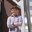Question-and-Answer Resource for the Building Energy Modeling Community
Get s tarted with the Help page

# cooling tower in free convection

OK, so the EnergyPlus engineering reference for (variable speed) cooling towers states that "The model will also account for tower performance in the “free convection” regime, when the tower fan is off but the water pump remains on." So, when I look at the performance curve (e.g. CoolTools), where DTA = f(TWB, DTR, fW, fA), with DTA = Approach, TWB = Wet Bulb Temperature, DTR = Range, fW = Water Flow Rate Ratio and fA = Air Flow Rate Ratio, and f() being a 35-coefficient curve, then in my mind, free convection would apply when fA = 0. Is that correct? Or would it be when fA=0.125, or some other Fraction of Tower Capacity in Free Convection Regime?

In addition, the fan power curve coefficients appear to be such that the fan power fraction is negative below fA=0.15, and positive above. But I suppose this has to to with fan VSD turn-own and fan cycling, rather than with free convection.

I am asking because I also tried to follow the logic in function void CalcVariableSpeedTower{} in EnergyPlus-8.7.0/src/CondenserLoopTowers.cc, which seems to complicate matters substantially beyond simple application of the f() curve?

For example, with constant fW=1, I would calculate the required DTR based on chiller operation and then, based on TWB, calculate the DTA for fA=0 to see what TCWS = TWB+DTA in free convection is. If it is less than the TCWSSP (setpoint), I am done. If it is more than TCWS, I would use Newton-Raphson to calculate fA from the f() curve such that DTA=TCWSSP-TWB for said required DTR. Of course, that leaves aside cycling and other finer points but it at least summarizes how I would want to go about free convection vs. forced convection.

Unfortunately, void CalcVariableSpeedTower{} is anything but that straight forward. Any enlightenment would be very much appreciated, especially since Input/Output Reference and Engineering Reference do not help me move on.

edit retag close merge delete

Sort by » oldest newest most votedThe calculation sequence looks like this:

Simulate tower at full speed
IF (Setpoint exceeded) THEN
Simulate tower in free convection mode
IF (Setpoint not met) THEN
Simulate tower at min fan speed
IF (Setpoint exceeded) THEN
Simulate tower cycling between min speed and free convection
ELSE
Find airflow ratio between min and max that meets setpoint
ENDIF
ENDIF
ENDIF


To simulate the tower in free convection mode, the program takes the results from the full speed simulation and multiplies the operating range by the Fraction of Tower Capacity in Free Convection Regime input field. Note that it does not run the tower curves at a "free convection airflow ratio" to do this. So the airflow ratio in the free convection mode is irrelevant. Also, if you are not careful, you could specify a free convection capacity that is higher than the capacity at min fan speed, which would give you some funny numbers.

The negative curve outputs probably has to do with the creators expecting that the curves wouldn't be used at such a low ratio. If your min fan speed is above 0.15, then you'll not get negative numbers. You're right in that it has nothing to do with free convection.

more

This is rather fascinating. Thank you for the insight. For the first "Setpoint exceeded", do you mean "TCWS(full speed) < TCWS(setpoint)"? For the second "Setpoint exceeded", do you mean "TCWS(minimum speed) < TCWS(setpoint)"? Also, what would be an example for "if you are not careful"?

1

Yes, that is correct on both points. An example of not being careful would be setting the Fraction of Tower Capacity in Free Convection Regime to something high like 0.5 with a min fan speed of 0.3. In this case, the outlet water temperature with the fan off would likely be less than with the fan at min speed. Following the calculation sequence, this would cause the tower to never cycle. If you use a more reasonable free convection capacity of something like 0-0.15, you won't have this issue.